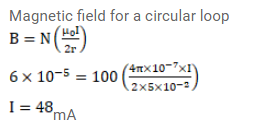# A current-carrying circular coil of 100 turns and radius

Question:

A current-carrying circular coil of 100 turns and radius $5.0 \mathrm{~cm}$ produces a magnetic field of $6.0 \times 10^{-5} \mathrm{~T}$ at its center. Find the value of the current.

Solution: